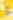# GROBNER DEFORMATIONS OF HYPERGEOMETRIC DIFFERENTIAL EQUATIONS

,

,

## Mutsumi Saito

Note moyenneIn recent years, new algorithms for dealing with rings of differential operators have been discovered and implemented. A main tool is the theory of Gröbner... Lire la suite
64,15 € Neuf

## Résumé

In recent years, new algorithms for dealing with rings of differential operators have been discovered and implemented. A main tool is the theory of Gröbner bases, which is re-examined here from the point of view of geometric deformations. Perturbation techniques have a long tradition in analysis ; Gröbner deformations of left ideals in the Weyl algebra are the algebraic analogue to classical perturbation techniques.
The algorithmic methods introduced in this book focus on the systems of multidimensional hypergeometric partial differential equations introduced by Gel'fand, Kapranov and Zelevinsky. The Gröbner deformation of these GKZ hypergeometric systems reduces problems concerning hypergeometric functions to questions about commutative monomial ideals, and thus leads to an unexpected interplay between analysis and combinatorics.
This book contains a number of original research results on holonomic systems and hypergeometric frictions, and it raises many open problems for future research in this rapidly growing area of computational mathematics.

## Sommaire

• BASIC NOTIONS
• Gröbner bases in the Weyl algebra
• Weight vectors and non-term orders
• The gauss hypergeometric equation
• Holonomic systems
• Integrals of products of linear forms
• SOLVING REGULAR HOLONOMIC SYSTEMS
• The Gröbner fan
• Semi-continuity of the holonomic rank
• Torus action and frobenius ideals
• Regular holonomic systems
• Canonical series solutions
• Construction of series solutions
• HYPERGEOMETRIC SERIES
• Gkz-hypergeometric ideal for generic parameters
• Standard pairs and triangulations
• The hypergeometric fan
• Logarithm-free hypergeometric series
• Lower bound for the holonomic rank
• Unimodular triangulations
• RANK VERSUS VOLUME
• The fake indicial ideal and a upper bound
• Hypergeometric functions from toric curves
• Koszul complexes and Cohen-Macaulay property
• Integer programming and parametric b-functions
• The exceptional hyperplane arrangement
• W-flatness
• INTEGRATION OF D-MODULES
• b-functions for holonomic D-ideals
• Computing restrictions
• Powers of polynomials
• Hypergeometric integrals
• Computing integrals
• Asymptotic expansions of hypergeometric integrals.

## Caractéristiques

• Date de parution
20/11/1999
• Editeur
• Collection
• ISBN
3-540-66065-8
• EAN
9783540660651
• Présentation
Relié
• Nb. de pages
255 pages
• Poids
0.445 Kg
• Dimensions
16,0 cm × 24,0 cm × 1,8 cm

## GROBNER DEFORMATIONS OF HYPERGEOMETRIC DIFFERENTIAL EQUATIONS est également présent dans les rayons

Decitre utilise des cookies pour vous offrir le meilleur service possible. En continuant votre navigation, vous en acceptez l'utilisation. En savoir plus OK
64,15 €